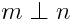# Perpendicular and relatively prime

Donald Knuth recommends using the symbol ⊥ between two numbers to indicate that they are relatively prime. For example:The symbol is denoted `\perp` in TeX because it is used in geometry to denote perpendicular lines. It corresponds to Unicode character U+27C2.

I mentioned this on TeXtip yesterday and someone asked for the reason for the symbol. I’ll quote Knuth’s original announcement and explain why I believe he chose that symbol.

When gcd(m, n) = 1, the integers m and n have no prime factors in common and we way that they’re relatively prime.

This concept is so important in practice, we ought to have a special notation for it; but alas, number theorists haven’t agreed on a very good one yet. Therefore we cry: Hear us, O Mathematicians of the World! Let us not wait any longer! We can make many formulas clearer by adopting a new notation now! Let us agree to write ‘mn ’, and to say “m is prime to n,” if m and n are relatively prime.

This comes from Concrete Mathematics. In the second edition, the text is on page 115. The book explains why some notation is needed, but it does not explain why this particular symbol.

[Correction: The book does explain the motivation for the symbol. The justification is in a marginal note and I simply overlooked it. The note says “Like perpendicular lines don’t have a common direction, perpendicular numbers don’t have common factors.”]

Here’s what I believe is the reason for the symbol.

Suppose m and n are two positive integers with no factors in common. Now pick numbers at random between 1 and mn. The probability of being divisible by m and n is 1/mn, the product of the probabilities of being divisible by m and n. This says that being divisible by m and being divisible by n are independent events. Also, independent events are analogous to perpendicular lines.  The analogy is made precise in this post where I show the connection between correlation and the law of cosines.

So in summary, the ideas of being relatively prime, independent, and perpendicular are all related, and so it makes sense to use a common symbol to denote each.

## 11 thoughts on “Perpendicular and relatively prime”

1. The image that naturally came to my mind was as follows: if we consider each prime number as a vector of a basis in an infinite-dimensional vector space, we can define each natural number as a vector holding the exponents of its prime decomposition, e.g. 21 = 3 x 7, so 21 = (0,1,0,1,0…), or 50 = 2 x 5 x 5, so 50 = (1,0,2,0…). Under such representation the inner product of two relatively prime numbers is clearly zero, i.e. they are perpendicular.

I wonder if a vector space can really be defined under such premises. Vector addition would be equivalent to natural number multiplication, so it would probably be necessary to include number of the form 1/N to make things work there. But I haven’t figured out what could scalar multiplication be in that context…

2. Jaime: I like your analogy with vector spaces. That makes a connection with orthogonality without having to invoke probability. As for scalar multiplication, I believe that would correspond to exponentiation.

What you’ve described is not quite a vector space because your scalars come from the integers and not from a field, but it’s like a vector space. I’m rusty on my algebra definitions, but I think what you’ve described may be a module.

3. I was going to make a similar comment but Jaime beat me to it…

Two vectors are perpendicular if and only if they have no components in common.

True, the analogy is imperfect. But if I asked you to define the “components” of a natural number, its prime factors might well spring to mind.

Even more simply… In common English, two concepts are called “orthogonal” if they have nothing in common.

4. I also think Jaime’s idea explains it in a way. Obviously the sequences of exponents do not define vector spaces but they do form modules. One may extend the concept of innerproduct and orthgonality to modules even though the generalization may not give nice duality. I haven’t seen such a theory but I am not an algebraist and you may find one somewhere.

5. Every now and then, I want to use that notation for independent or at least uncorrelated variables, and check to make sure it’s already in use. I’m always a little sad to find it isn’t, and I’ll have to spell the words out.

6. Sesqu: I’d also like to use this notation in probability, but it wouldn’t be understood. I think it may have gained more acceptance in number theory.

7. Chris Barts

Also, the vertical bar ‘m | n’ means ‘m divides n’, so the symbol Knuth proposes is a simple modification of that.

8. I find the vector analogy works better if you use logs instead. A=B*C*D*D, then log(A) = log(B)+log(C)+2log(D). The basis vectors are then simply the logs of the primes. You may need to extend it to include log(1) on the first row as well, I suspect!

Interestingly, with this version in mind, it shows why computing the factors of P+2 is not straightforward – log(P+2) is not separable into some combination of log(P) and log(2), so neither are the vectors.

9. lucy alquiler departamentos miami

Of couse, two vectors are perpendicular if and only if they have no components in common.

10. @Chris: Knuth et al actually use mn for ‘m divides n’. :) I also like the idea of using asymmetric symbols for asymmetric relations.

Joao

11. Alan Shaw

One of my professors at the Courant Institute, Malcolm Harrison, used to use the phrase “orthogonal issues” when someone brought up two things that had nothing to do with one another.